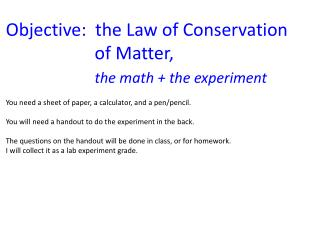# Objective: the Law of Conservation of Matter, the math + the experiment - PowerPoint PPT PresentationDownload PresentationObjective: the Law of Conservation of Matter, the math + the experiment

Objective: the Law of Conservation of Matter, the math + the experimentDownload Presentation## Objective: the Law of Conservation of Matter, the math + the experiment

- - - - - - - - - - - - - - - - - - - - - - - - - - - E N D - - - - - - - - - - - - - - - - - - - - - - - - - - -
##### Presentation Transcript

1. Objective: the Law of Conservation of Matter, the math + the experiment You need a sheet of paper, a calculator, and a pen/pencil. You will need a handout to do the experiment in the back. The questions on the handout will be done in class, or for homework. I will collect it as a lab experiment grade.

2. The Law of Conservation of Matter Matter cannot be created or destroyed in any chemical reaction (or in a physical phase change). Iron + oxygen yields Iron oxide Iron and oxygen are the __________________ Iron oxide is the ______________________ The mass of the _____________ equals the mass of the __________

3. 223 g Iron + 96 g Oxygen ______ Iron Oxide 4 g hydrogen + X g oxygen 36 grams water

4. 223 g Iron + 96 g Oxygen 319g Iron Oxide 223 g + 96 g = 319 g Iron Oxide 4 g hydrogen + 32 g oxygen 36 grams water 4 g + X g = 36 g X = 32 g water

5. 8 g hydrogen + 64 g oxygen make how many g water? ______ ____ g hydrogen + 28 g nitrogen 34 g ammonia (NH3)

6. 8 g hydrogen + 64 g oxygen make how many g water? 72 g water ____ g hydrogen + 28 g nitrogen 34 g ammonia (NH3) X + 28 = 34 X = 6 grams of hydrogen

7. In two minutes we are getting up. Follow along with your handout now. Obtain 2 small jars, one bigger than the other. Get a lid for the larger jar. Fill the small jar half way with the solution labeled: silver nitrate solution Fill the larger jar about one quarter full with this solution: sodium phosphate solution Mass both jars and cap on the scale all at once. Write down their masses. Very gingerly, slip the small jar into the larger without spilling a drop. Re-cap the big jar Very carefully mass the whole system together, make sure that the total mass equals the mass of the two jars separately. If not, tell me. Flip the jars over twice and watch the chemical reaction. The word equation for what happened is: Mass the jars again, what is their mass with these new substances inside.

8. FLIP!

9. Handout out questions… Name the two solutions that you mixed. Name the two new substances that formed What does aqueous mean? What does soluble mean? What does insoluble mean? What is a precipitate? What is the start mass and end mass difference? State the Law of Conservation of Matter. Describe the little experiment in two or three sentences, use descriptions.